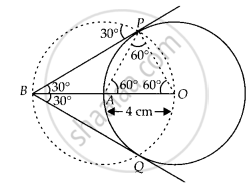# Draw a circle of radius 4 cm. Construct a pair of tangents to it, the angle between which is 60º. Also justify the construction. Measure the distance between the centre of the circle and th - Mathematics

Diagram
Sum

Draw a circle of radius 4 cm. Construct a pair of tangents to it, the angle between which is 60º. Also justify the construction. Measure the distance between the centre of the circle and the point of intersection of tangents.

#### Solution

Steps of construction:

1. Take a point O on the plane of the paper and draw a circle of radius OA = 4 cm.

2. Produce OA to B such that OA = AB = 4 cm.

3. Taking A as the centre draw a circle of radius AO = AB = 4 cm. Suppose it cuts the circle drawn in step 1 at P and Q.

4. Join BP and BQ to get desired tangents.

Justification:

In ∆OAP, we have

OA = OP = 4 cm  ......(Radius)

Also, AP = 4 cm  .......(∵ Radius of circle with centre A)

∴ ∆OAP is equilateral

⇒ ∠PAO = 60°

⇒ ∠BAP = 120°In ∆BAP, we have

BA = AP and ∠BAP = 120°

∴ ∠ABP = ∠APB = 30° ⇒ ∠PBQ = 60°

Is there an error in this question or solution?
Chapter 10: Construction - Exercise 10.4 [Page 118]

#### APPEARS IN

NCERT Exemplar Class 10 Mathematics
Chapter 10 Construction
Exercise 10.4 | Q 6 | Page 118
Share